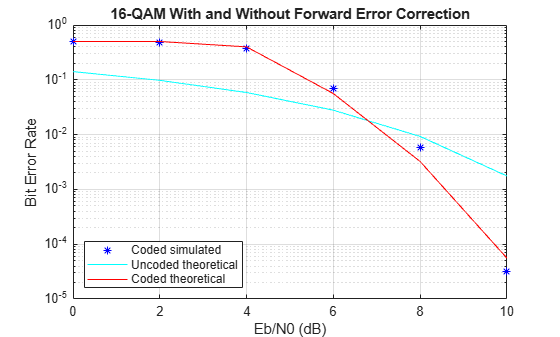Rate 2/3 Convolutional Code in AWGN

This example generates a bit error rate versus Eb/No curve for a link that uses 16-QAM modulation and a rate 2/3 convolutional code in AWGN.

Set the modulation order, and compute the number of bits per symbol.

M = 16;
k = log2(M);

Create a trellis for a rate 2/3 convolutional code. Set the traceback and code rate parameters.

trellis = poly2trellis([5 4],[23 35 0; 0 5 13]);
traceBack = 16;
codeRate = 2/3;

Create a convolutional encoder and its equivalent Viterbi decoder.

convEncoder = comm.ConvolutionalEncoder('TrellisStructure',trellis);
vitDecoder = comm.ViterbiDecoder('TrellisStructure',trellis, ...
'InputFormat','Hard','TracebackDepth',traceBack);

Create an error rate object. Set the receiver delay to twice the traceback depth, which is the delay through the decoder.

Set the range of Eb/No values to be simulated. Initialize the bit error rate statistics matrix.

ebnoVec = 0:2:10;
errorStats = zeros(length(ebnoVec),3);

Simulate the link by following these steps:

• Generate binary data.

• Encode the data with a rate 2/3 convolutional code.

• 16-QAM modulate the encoded data, configure bit inputs and unit average power.

• Pass the signal through an AWGN channel.

• 16-QAM demodulate the received signal configure bit outputs and unit average power.

• Decode the demodulated signal by using a Viterbi decoder.

• Collect the error statistics.

for m = 1:length(ebnoVec)
snr = ebnoVec(m) + 10*log10(k*codeRate);
while errorStats(m,2) <= 100 && errorStats(m,3) <= 1e7
dataIn = randi([0 1],10000,1);
dataEnc = convEncoder(dataIn);
txSig = qammod(dataEnc,M, ...
'InputType','bit','UnitAveragePower',true);
rxSig = awgn(txSig,snr);
demodSig = qamdemod(rxSig,M, ...
'OutputType','bit','UnitAveragePower',true);
dataOut = vitDecoder(demodSig);
errorStats(m,:) = errorRate(dataIn,dataOut);
end
reset(errorRate)
end

Compute the theoretical BER vs. Eb/No curve for the case without forward error correction coding.

berUncoded = berawgn(ebnoVec','qam',M);

Plot the BER vs. Eb/No curve for the simulated coded data and the theoretical uncoded data. At higher Eb/No values, the error correcting code provides performance benefits.

semilogy(ebnoVec,[errorStats(:,1) berUncoded])
grid
legend('Coded','Uncoded')
xlabel('Eb/No (dB)')
ylabel('Bit Error Rate')